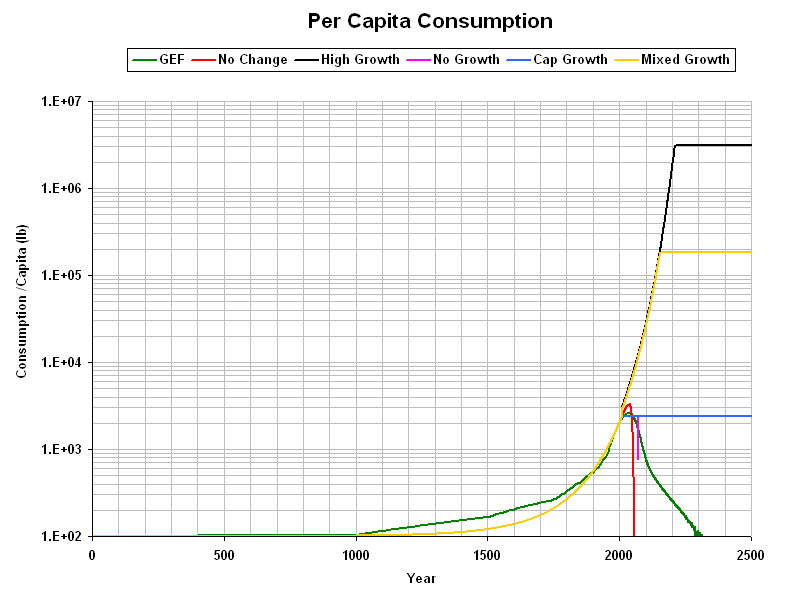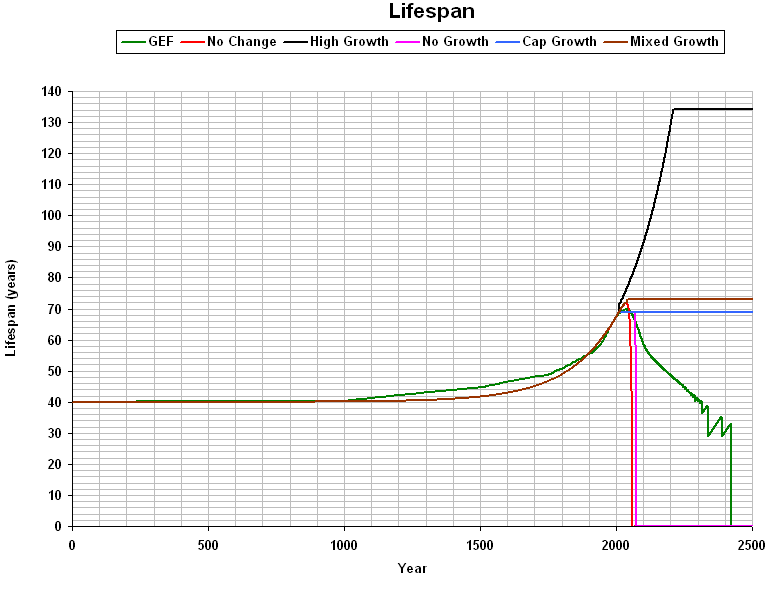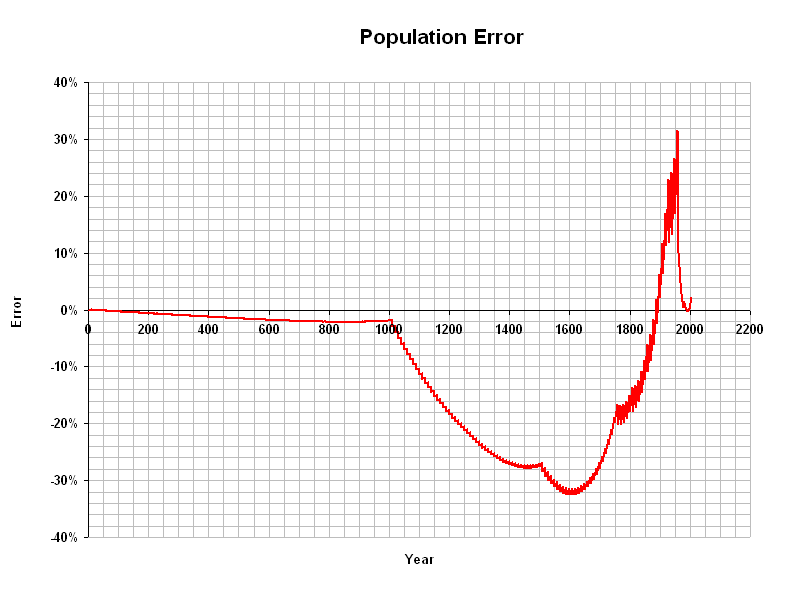Combined Population Model

The combined population model, like the speed model, assumes that population is proportional to the square root of annual resource consumption. The consumption model's correlation between the fraction of remaining resources and population is incorporated into the mathematical description of consumption, allowing for empirical determination of the associated exponent of that ratio.

Version 1 of the model is defined as follows:

Constants: a, b, d, g, h, j, k

Time: T = year

Mass of maximum (non-renewable) resources: Mmax = Maximum possible resources

Mass of starting resources: Mstart = Resources at time Tstart

Mass of remaining resources: M(T)

Rate of resource growth: Mrate

Resource ratio: Mratio = M(T)/M(T-1)

Consumption: C = a*[Mratio^b]*T^EXP(d*T^g+h)

Renewable resources: R

M(T) = M(T-1)*[1+Mrate]-[C-R]*[C-R>0]

Population: P = j*C^.5

Speed: S = k*C

Following is the result of a manual curve-fit to the data for several scenarios. "No Change" continues business-as-usual, with no change is resources or consumption behavior. "High Growth" continues the historical growth in consumption, limited only by speed of resource access (the speed of light) and maximum resources equal to one Earth mass. "No Growth" sets consumption constant beginning in 2010. "Cap Growth" increases capacity (resources that are renewable) to match consumption in 2010 while keeping consumption constant. "Mixed Growth" increases both non-renewable and renewable resources to one Earth mass while keeping consumption constant. The consumption model's ecological resources projection ("GEF") is included for comparison, which includes historical data to 2005.Per capita consumption is given below, assuming a starting value of 100 pounds:The following graph gives the average speed of resource movement (per the speed model) for each of the scenarios:The amount of resources remaining is plotted below:

The cumulative distance that might have been traveled since 0 A.D. is shown below using half the speed for each year. Units are astronomical units (AU), where 1 AU is the mean distance from Earth to the Sun.Following is the projected life expectancy (lifespan), based on the Third Law of Consumption:Following is the error in projections of population over time (for the "No Change" scenario):